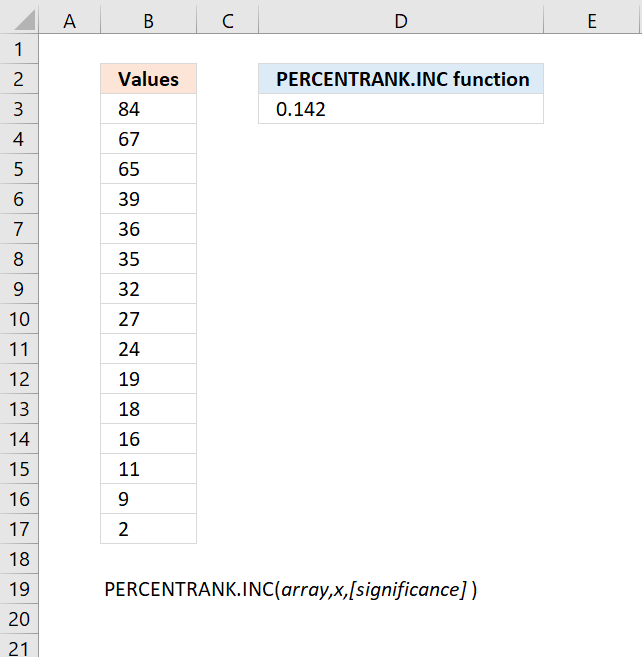Author: Oscar Cronquist Article last updated on May 07, 2022The PERCENTRANK.INC function calculates the percent rank of a given number compared to the whole data set. The image above shows 15 numbers in column B. 2 numbers are less than 11 (second argument) and 12 numbers are larger than 11.

2/(2+12) = 0.142 (approx 3 digits)

This function was introduced in Excel 2010 and has replaced the PERCENTRANK function.

Formula in cell C7:

=PERCENTRANK.INC(B3:B17,11)

### Excel Function Syntax

PERCENTRANK.INC(arrayx[significance])

### Arguments

 array Required. A parameter which determines the shape of the distribution. x Required. A parameter which determines the shape of the distribution. [significance] Optional. Lower bound, default value 0 (zero).